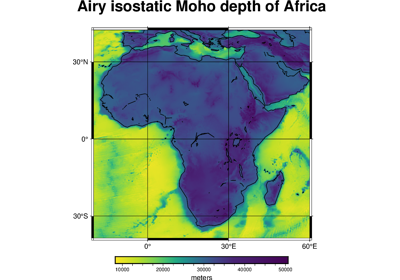# harmonica.isostatic_moho_airy¶

harmonica.isostatic_moho_airy(basement, layers=None, density_crust=2800.0, density_mantle=3300.0, reference_depth=30000.0)[source]

Calculate the isostatic Moho depth using Airy’s hypothesis.

Take the height of the crystalline basement and optional additional layers located on top of it. Each layer must be specified through its vertical thickness and its corresponding density. Return the depth to the Mohorovicic discontinuity (crust-mantle interface).

Parameters
• basement (float or array) – Height of the crystalline basement in meters. It usually refer to topography and bathymetry height without sediment cover. When considering sedimentary basins, it refers to crystalline basement (topography/bathymetry minus sediment thickness).

• layers (dict (optional)) – Dictionary that contains information about the thickness and density of the layers located above the basement. For each layer, a single item should be created: its key will be the layer name as a str and its values must be tuples containing the layer thickness in meters and the layer density (in $$kg/m^3$$) in that order. Thicknesses and densities can be floats or arrays. If None, no layers will be considered. Default as None.

• density_crust (float or array (optional)) – Density of the crust in $$kg/m^3$$.

• density_mantle (float or array (optional)) – Mantle density in $$kg/m^3$$.

• reference_depth (float or array (optional)) – The reference Moho depth ($$H$$) in meters.

Returns

moho_depth (float or array) – The isostatic Moho depth in meters.

Notes

According to the Airy hypothesis of isostasy, rock equivalent topography above sea level is supported by a thickening of the crust (a root) while rock equivalent topography below sea level is supported by a thinning of the crust (an anti-root). This assumption is usuallySchematic of isostatic compensation following the Airy hypothesis.

The relationship between the rock equivalent topography ($$r_{et}$$) and the root thickness ($$r$$) is governed by mass balance relations and can be found in classic textbooks like [TurcotteSchubert2014] and [Hofmann-WellenhofMoritz2006].

Compress all layers’ mass above basement ($$h$$) into rock equivalent topography [Balmino1973] :

$r_{et} = h + \sum\limits_{i=1}^N \frac{\rho_{i}}{\rho_{c}} t_{i}$

Based on rock equivalent topography, the root is calculated as:

$r = \frac{\rho_{c}}{\rho_m - \rho_{c}} r_{et}$

in which $$r_{et}$$ is the rock equivalent topography , $$\rho_m$$ is the density of the mantle, and $$\rho_{c}$$ is the density of the crust.

The computed root thicknesses will be added to the given reference Moho depth ($$H$$) to arrive at the isostatic Moho depth. Use reference_depth=0 if you want the values of the root thicknesses instead.

Examples

Simple model of continental topography with a sedimentary basin on top

>>> # Define crystalline basement height (in meters)
>>> basement = 1200
>>> # Define a layer of sediments with a thickness of 200m
>>> sediments_thickness = 200
>>> sediments_density = 2300
>>> # Get depth of the Moho following Airy's isostatic hypothesis
>>> moho_depth = isostatic_moho_airy(
...     basement,
...     layers={"sediments": (sediments_thickness, sediments_density)}
... )
>>> moho_depth
37640.0


Simple model of oceanic sedimentary basin

>>> # Define bathymetry (in meters)
>>> bathymetry = -3000
>>> # Define a layer of sediments with a thickness of 400m
>>> sediments_thickness = 400
>>> sediments_density = 2200
>>> # Define a layer for the oceanic water
>>> water_thickness = abs(bathymetry)
>>> water_density = 1040
>>> # Get depth of the Moho following Airy's isostatic hypothesis
>>> moho_depth = isostatic_moho_airy(
...     bathymetry - sediments_thickness,
...     layers={
...         "sediments": (sediments_thickness, sediments_density),
...         "water": (water_thickness, water_density),
...     }
... )
>>> moho_depth
18960.0


## Examples using harmonica.isostatic_moho_airy¶